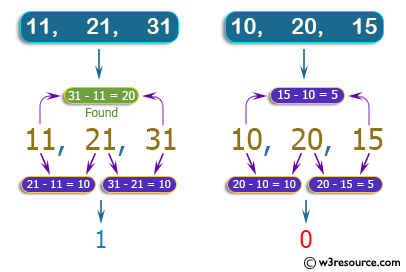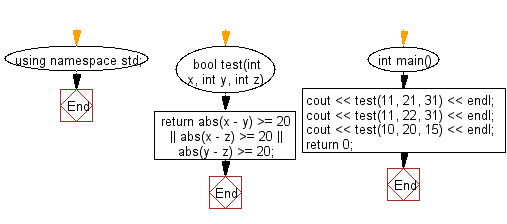﻿ C++ : If one of 3 integers is 20 or more, less than others# C++ Exercises: Check three given integers and return true if one of them is 20 or more less than one of the others

## C++ Basic Algorithm: Exercise-48 with Solution

Write a C++ program to check three given integers and return true if one of them is 20 or more less than one of the others.

Sample Solution:

C++ Code :

``````#include <iostream>
using namespace std;

bool test(int x, int y, int z)
{
return abs(x - y) >= 20 || abs(x - z) >= 20 ||
abs(y - z) >= 20;
}

int main()
{
cout << test(11, 21, 31) << endl;
cout << test(11, 22, 31) << endl;
cout << test(10, 20, 15) << endl;
return 0;
}
``````

Sample Output:

```1
1
0
```

Pictorial Presentation:Flowchart:C++ Code Editor: